# Chapter 2 Ordinary Differential Equations

## 2.1  Reduction to first-order

An ordinary differential equation involves just one independent variable, $x$, and a dependent variable $y$. Obviously it involves derivatives of the dependent variable like $\frac{\mathit{dy}}{\mathit{dx}}$. The highest order differential, i.e. the term $\frac{{d}^{N}y}{{\mathit{dx}}^{N}}$ with the largest value of $N$ appearing in the equation, defines the order $N$ of the equation. So the most general ODE of order $N$ can be written such that the $N$th order derivative is equal to a function of all the lower order derivatives and the independent variable $x$:
 $\frac{{d}^{N}y}{{\mathit{dx}}^{N}}=f\left(\frac{{d}^{N-1}y}{{\mathit{dx}}^{N-1}},\frac{{d}^{N-2}y}{{\mathit{dx}}^{N-2}},\dots ,\frac{\mathit{dy}}{\mathit{dx}},y,x\right)$ $\left(2.1\right)$
Such an ordinary differential equation of order $N$ in a single dependent variable, $y$, can always be reduced to a set of simultaneous coupled first order equations involving $N$ dependent variables. The simplest way to do this is to use a natural notation to denote by ${y}^{\left(i\right)}$ the $i$th derivative:
 $\frac{{d}^{i}y}{{\mathit{dx}}^{i}}={y}^{\left(i\right)}\mathrm{ }i=1,2,\dots ,N-1.$ $\left(2.2\right)$
When combined with the original equation, the total system can be written as a first-order vector differential equation whose components are
 $\frac{d}{\mathit{dx}}{y}^{\left(i\right)}={f}_{i}\left({y}^{\left(N-1\right)},{y}^{\left(N-2\right)},\dots ,{y}^{\left(1\right)},{y}^{\left(0\right)},x\right),\mathrm{ }i=0,1,\dots ,N-1$ $\left(2.3\right)$
(where for notational consistency ${y}^{\left(0\right)}=y$). Explicitly in vector form:
 $\frac{d}{\mathit{dx}}\left(\begin{array}{c}\hfill {y}^{\left(0\right)}\hfill \\ \hfill {y}^{\left(1\right)}\hfill \\ \hfill :\hfill \\ \hfill {y}^{\left(N-1\right)}\hfill \end{array}\right)=\left(\begin{array}{c}\hfill {f}_{0}\hfill \\ \hfill {f}_{1}\hfill \\ \hfill :\hfill \\ \hfill {f}_{N-1}\hfill \end{array}\right)=\left(\begin{array}{c}\hfill {y}^{\left(1\right)}\hfill \\ \hfill {y}^{\left(2\right)}\hfill \\ \hfill :\hfill \\ \hfill f\left({y}^{\left(N-1\right)},{y}^{\left(N-2\right)},\dots ,{y}^{\left(1\right)},{y}^{\left(0\right)},x\right)\hfill \end{array}\right).$ $\left(2.4\right)$
Recognizing that the combined simultanous vector system of dimension $N$ with first-order derivatives, is equivalent to a single scalar equation of order $N$, we often say that the order of the coupled vector system is still $N$. (Sorry if that seems confusing. In practice you get the hang of it.)
This is formal mathematics and applies to all equations, but precisely such a set of coupled first-order equations will often also arise directly in the formulation of the practical problem we are trying to solve. Suppose we are trying to track the position of a fluid element in a three dimensional steady flow. If we know the fluid velocity $\mathbit{v}$ as a function of position $\mathbit{v}\left(\mathbit{x}\right)$, then the equation of the track of a fluid element, i.e. the path followed by the element as it moves in time $t$, is
 $\frac{d}{\mathit{dt}}\mathbit{x}=\mathbit{v}.$ $\left(2.5\right)$
This is the equation we must solve to find a fluid streamline. It is of just the same form we derived by order reduction. Such a history of position as a function of time is called generically an orbit. Here the independent variable is $t$, and the dependent variable is $\mathbit{x}$. The vector $\mathbit{v}$ plays the role of the functions ${f}_{i}$.
Orbits may not be just in space, they may be in higher-dimensional phase-space. For example (see Fig 2.1) to find the orbit of a charged particle (e.g. proton) of mass ${m}_{p}$ and charge $e$ moving at velocity $\mathbit{v}$ in a uniform magnetic field $\mathbit{B}$, we observe that it is subject to a force $e\mathbit{v}×\mathbit{B}$. In the absence of any other forces, it has an equation of motion
 $\frac{d}{\mathit{dt}}\mathbit{v}=\frac{e}{{m}_{p}}\mathbit{v}×\mathbit{B}=\mathbit{f}\left(\mathbit{v}\right),$ $\left(2.6\right)$
in which the acceleration depends upon the velocity. This is a first order vector differential equation, in three dimensions, where $t$ plays the role of the independent variable, and the dependent variable is the vector velocity $\mathbit{v}$. The vector acceleration $\mathbit{f}$ which is the vector derivative function depends upon all the components of $\mathbit{v}$.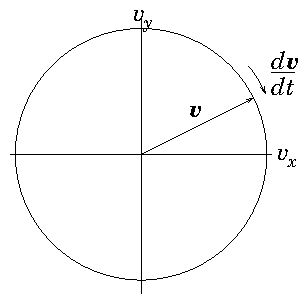Figure 2.1: Orbit of the velocity of a particle moving in a uniform magnetic field is a circle in velocity-space perpendicular to the field ($B=B\stackrel{^}{\mathbit{z}}$ here).
If, for our proton orbit, $\mathbit{B}$ is not uniform, but varies with position, then we need to know both position $\mathbit{x}$, and velocity $\mathbit{v}$ at all times along the orbit to solve it. The system then involves six-dimensional vectors consisting of the components of $\mathbit{x}$ and $\mathbit{v}$:

 $\frac{d}{\mathit{dt}}\left(\begin{array}{c}\hfill {x}_{1}\hfill \\ \hfill {x}_{2}\hfill \\ \hfill {x}_{3}\hfill \\ \hfill {v}_{1}\hfill \\ \hfill {v}_{2}\hfill \\ \hfill {v}_{3}\hfill \end{array}\right)=\left(\begin{array}{c}\hfill {v}_{1}\hfill \\ \hfill {v}_{2}\hfill \\ \hfill {v}_{3}\hfill \\ \hfill \left({v}_{2}{B}_{3}-{v}_{3}{B}_{2}\right)e/{m}_{p}\hfill \\ \hfill \left({v}_{3}{B}_{1}-{v}_{1}{B}_{3}\right)e/{m}_{p}\hfill \\ \hfill \left({v}_{1}{B}_{2}-{v}_{2}{B}_{1}\right)e/{m}_{p}\hfill \end{array}\right).$ $\left(2.7\right)$
Very often, to find analytic solutions it feels more natural to eliminate some of the dependent variables with the result that the order of the ODE is raised. So, for example for a uniform magnetic field in the $3$-direction, the dynamics perpendicular to it separate out into
 $\frac{d}{\mathit{dt}}{v}_{1}=\mathit{\Omega }{v}_{2}, \frac{d}{\mathit{dt}}{v}_{2}=-\mathit{\Omega }{v}_{1}\mathrm{ }⇒\mathrm{ }\frac{{d}^{2}{v}_{1}}{{\mathit{dt}}^{2}}=-{\mathit{\Omega }}^{2}{v}_{1}, \frac{{d}^{2}{v}_{2}}{{\mathit{dt}}^{2}}=-{\mathit{\Omega }}^{2}{v}_{2}.$ $\left(2.8\right)$
(writing $\mathit{\Omega }=\mathit{eB}/{m}_{p}$). The second-order uncoupled equations are familiar to us as simple harmonic oscillator equations, having solutions like $\mathrm{cos}\mathit{\Omega }t$ and $\mathrm{sin}\mathit{\Omega }t$. So they are easier to solve analytically. But the original first-order equations, even though they are coupled are far easier to solve numerically. So we don't generally do the elimination in computational solutions.

## 2.2  Numerical Integration of Initial Value Problem

### 2.2.1  Explicit Integration

Now we consider how in practice to solve a first-order ODE in which all the boundary conditions are imposed at the same position in the independent variable. Such boundary conditions constitute what is called an "initial value problem". We start integrating forward in the independent variable (e.g. time or space) from a place where the initial values are specified. To simplify the discussion we will consider a single (scalar) dependent variable $y$, but note that the generalization to a vector of dependent variables is usually immediate, so the treatment is fine for higher order equations that have been reduced to vectorial first-order form.
In general, numerical solution of differential equations requires us to represent the solution, which is usually continuous, in a discrete manner where the values are given at a series of points rather than continuously. See Fig. 2.2.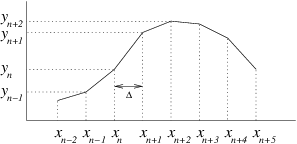Figure 2.2: Illustrating finite difference representation of a continuous function.
The natural way to discretize the derivative is to write
 $\frac{\mathit{dy}}{\mathit{dx}}\approx \frac{{y}_{n+1}-{y}_{n}}{{x}_{n+1}-{x}_{n}}=f\left(y,x\right),$ $\left(2.9\right)$
where the index $n$ denotes the value at the $n$th discrete step, and therefore
 ${y}_{n+1}={y}_{n}+f\left(y,x\right)\left({x}_{n+1}-{x}_{n}\right).$ $\left(2.10\right)$
This equation tells us how $y$ changes from one step to the next. Starting from an initial position we can step discretely as far as we like, choosing the size of the independent variable step $\left({x}_{n+1}-{x}_{n}\right)=\mathit{\Delta }$ appropriately.
A question that arises, though, is what to use for $x$ and $y$ inside the derivative function $f\left(y,x\right)$. The $x$ value can be chosen more or less at will${}^{13}$ but before we've actually made the step, we don't know where we are going to end up in $y$, so we can't easily decide where in $y$ to evaluate $f$. The easiest answer, but not generally the best, is to recognize that at any point in stepping from $n$ to $n+1$ along the orbit, we already have the value ${y}_{n}$. So we could just use $f\left({y}_{n},{x}_{n}\right)$. This choice is said to be "explicit", and is sometimes called the Euler method. The reason why this method is not the best is because it tends have poor accuracy and poor stability.

### 2.2.2  Accuracy and Runge-Kutta Schemes

To illustrate the problem of accuracy, consider the derivative function $f$ expanded as a Taylor series about the ${x}_{n},{y}_{n}$ position, writing $x-{x}_{n}=\mathit{\delta }x$, $y-{y}_{n}=\mathit{\delta }y$. The derivative function $f$ is a function of both $x$ and $y$. However, the solution for the orbit can be written $y=y\left(x\right)$. Therefore the function evaluated on the orbit, $f\left(y\left(x\right),x\right)$, is a function only of $x$, and we can write its (total) derivative as $\frac{\mathit{df}}{\mathit{dx}}$. The Taylor expansion of this function is simply${}^{14}$
 $f\left(y\left(x\right),x\right)=f\left({y}_{n},{x}_{n}\right)+\frac{{\mathit{df}}_{n}}{\mathit{dx}}\mathit{\delta }x+\frac{{d}^{2}{f}_{n}}{{\mathit{dx}}^{2}}\frac{\mathit{\delta }{x}^{2}}{2!}+O\left(\mathit{\delta }{x}^{3}\right)$ $\left(2.11\right)$
We use the notation ${\mathit{df}}_{n}/\mathit{dx}$ (etc.) to indicate values evaluated at position $n$. If we substitute this Taylor expansion for $f$ into the differential equation we are trying to solve, $\mathit{dy}/\mathit{dx}=d\mathit{\delta }y/d\mathit{\delta }x=f$, and integrate term by term we get the exact solution of the differential equation.
 $\mathit{\delta }y={f}_{n}\mathit{\delta }x+\frac{{\mathit{df}}_{n}}{\mathit{dx}}\frac{\mathit{\delta }{x}^{2}}{2!}+\frac{{d}^{2}{f}_{n}}{{\mathit{dx}}^{2}}\frac{\mathit{\delta }{x}^{3}}{3!}+O\left(\mathit{\delta }{x}^{4}\right)$ $\left(2.12\right)$
We subtract from it whatever the finite difference approximate equation is. In the case of (2.10) it is $\mathit{\delta }{y}^{\left(1\right)}={f}_{n}\mathit{\delta }x$, and we find that the error in ${y}_{n+1}$ is
 $\mathit{\delta }y-\mathit{\delta }{y}^{\left(1\right)}=\mathit{\epsilon }=\frac{{\mathit{df}}_{n}}{\mathit{dx}}\frac{\mathit{\delta }{x}^{2}}{2!}+\frac{{d}^{2}{f}_{n}}{{\mathit{dx}}^{2}}\frac{\mathit{\delta }{x}^{3}}{3!}+O\left(\mathit{\delta }{x}^{4}\right).$ $\left(2.13\right)$
This tells us that the explicit Euler difference scheme is accurate only to first-order in the size of the step $\mathit{\delta }x$ (when the first derivative of $f$ is non-zero) because an error of order $\mathit{\delta }{x}^{2}$ is present. That means if we make the step a factor of 2 smaller, the cumulative error, when integrating over a set total distance, gets smaller by (approximately) a factor of 2. (Because each step's error is 4 times smaller, but there are twice as many steps.) That's not very good. We would have to take very small steps, $\mathit{\delta }x$, to get good accuracy.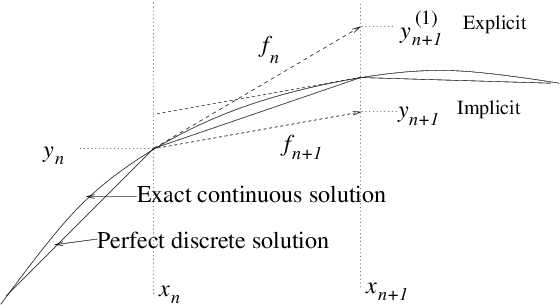Figure 2.3: Optional steps using derivative function evaluated at $n$ or $n+1$.
We can do better. Our error arose because we approximated the derivative function by using its value only at ${x}_{n}$. But once we've moved to the next position, and know (with some inaccuracy) the value ${y}_{n+1}$ and hence ${f}_{n+1}$ there, we can evaluate better the $f$ we should have used. This process is illustrated in Fig. 2.3. In fact, by substitution from eq. (2.11) it is easy to see that if we use, instead, the advancing equation
 $\mathit{\delta }y=\frac{1}{2}\left({f}_{n}+{f}_{n+1}^{\left(1\right)}\right)\mathit{\delta }x,$ $\left(2.14\right)$
where ${f}_{n+1}^{\left(1\right)}=f\left({y}_{n+1}^{\left(1\right)},{x}_{n+1}\right)$ is the value of $f$ obtained at the end of our first (explicit Euler) advance ${y}_{n+1}^{\left(1\right)}={y}_{n}+{f}_{n}\mathit{\delta }x$, then we would obtain for our approximate advancing scheme
 $\mathit{\delta }y={f}_{n}\mathit{\delta }x+\frac{{\mathit{df}}_{n}}{\mathit{dx}}\frac{\mathit{\delta }{x}^{2}}{2!}+O\left(\mathit{\delta }{x}^{3}\right),$ $\left(2.15\right)$
which now agrees with the first two terms of the full exact expansion (2.12), and whose error, in comparison with that expression, is now of third order, rather than second. This second-order accurate scheme gives cumulative errors proportional to $\mathit{\delta }{x}^{2}$ and so converges much more quickly as we shorten the step length.
The reason we obtained a more accurate step was that we used a more accurate value for the average (over the step) of the derivative function. It is straightforward to improve the average even more, so as to obtain even higher order accuracy. But to do that requires us to obtain estimates of the derivative function part way through the step as well as at the ends. That's because we need to estimate the first and second derivatives of $f$.
A Runge-Kutta method consists of taking a series of steps, each one of which uses the estimate of the derivative function obtained from the previous one, and then taking some weighted average of their derivatives.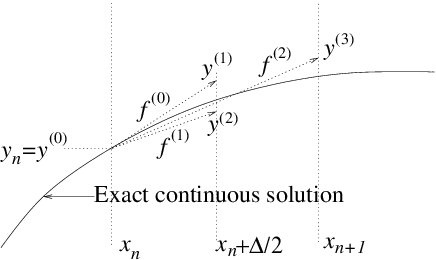Figure 2.4: Runge-Kutta fourth-order scheme with four partial steps, evaluates the derivative function ${f}^{\left(k\right)}$ with $k=0,1,2,3$, at four places $\left({x}_{k},{y}_{k}\right)$, each determined by extrapolation along the previous derivative.
Specifically, the fourth-order (accurate) Runge-Kutta scheme, which is by far the most popular and is illustrated in Fig. 2.4, uses:
 $\begin{array}{ccc}\multicolumn{1}{c}{{f}^{\left(0\right)}}& =\hfill & f\left({y}_{n},{x}_{n}\right)\hfill \\ \multicolumn{1}{c}{{f}^{\left(1\right)}}& =\hfill & f\left({y}_{n}+{f}^{\left(0\right)}\frac{\mathit{\Delta }}{2},{x}_{n}+\frac{\mathit{\Delta }}{2}\right)\hfill \\ \multicolumn{1}{c}{{f}^{\left(2\right)}}& =\hfill & f\left({y}_{n}+{f}^{\left(1\right)}\frac{\mathit{\Delta }}{2},{x}_{n}+\frac{\mathit{\Delta }}{2}\right)\hfill & \hfill \left(2.16\right)\\ \multicolumn{1}{c}{{f}^{\left(3\right)}}& =\hfill & f\left({y}_{n}+{f}^{\left(2\right)}\mathit{\Delta },{x}_{n}+\mathit{\Delta }\right),\hfill \end{array}$

in which two steps are to the half-way point $\frac{\mathit{\Delta }}{2}$. Then the following combination
 ${y}_{n+1}={y}_{n}+\left(\frac{{f}^{\left(0\right)}}{6}+\frac{{f}^{\left(1\right)}}{3}+\frac{{f}^{\left(2\right)}}{3}+\frac{{f}^{\left(3\right)}}{6}\right)\mathit{\Delta }+O\left({\mathit{\Delta }}^{5}\right),$ $\left(2.17\right)$
gives an approximation accurate to fourth-order${}^{15}$
The Runge-Kutta method costs more computation per step, because it requires four evaluations of the function $f\left(y,x\right)$, rather than just one. But that is often more than compensated by the ability to take larger steps than with the Euler method for the same accuracy.

### 2.2.3  Stability

The second, and possibly more important, weakness of explicit integration is in respect of stability. Consider a linear differential equation
 $\frac{\mathit{dy}}{\mathit{dx}}=-ky,$ $\left(2.18\right)$
where $k$ is a positive constant. This of course has the solution $y={y}_{0}\mathrm{exp}\left(-kx\right)$. But suppose we integrate it numerically using the explicit scheme
 ${y}_{n+1}={y}_{n}+f\left({y}_{n},{x}_{n}\right)\left({x}_{n+1}-{x}_{n}\right)={y}_{n}\left(1-k\mathit{\Delta }\right).$ $\left(2.19\right)$
This finite difference equation has the solution
 ${y}_{n}={y}_{0}\left(1-k\mathit{\Delta }{\right)}^{n},$ $\left(2.20\right)$
as may be verified by the simple observation that ${y}_{n+1}/{y}_{n}=\left(1-k\mathit{\Delta }\right)$. (This ratio is called the amplification factor.) If $k\mathit{\Delta }$ is a small number, then no difficulties will arise, and our scheme will produce approximately correct results. However, a choice $k\mathit{\Delta }>2$ compromises not only accuracy, but also stability. The resulting solution has alternating sign; it oscillates; but also its magnitude increases with $n$ and will tend to infinity at large $x$. It has become unstable, as illustrated in Fig. 2.5.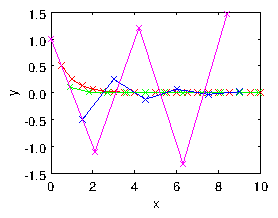Figure 2.5: Explicit numerical integration of eq. (2.18), using eq.  (2.19) leads to an oscillatory instability if the step length is too long. Four step lengths are shown $k\mathit{\Delta }=0.5,0.9,1.5,2.1$.
In general, an explicit discrete advancing scheme requires the step in the independent variable to be less than some value (in this case $2/k$) in order to achieve stability.
An implicit advancing scheme, by contrast, is one in which the value of the derivative used to advance the variable is taken at the end of the step rather than at the beginning. For our example equation, this would be a numerical scheme of the form
 ${y}_{n+1}={y}_{n}+f\left({y}_{n+1},{x}_{n+1}\right)\left({x}_{n+1}-{x}_{n}\right)={y}_{n}-k{y}_{n+1}\mathit{\Delta }.$ $\left(2.21\right)$
It is easy to rearrange this equation into
 ${y}_{n+1}\left(1+k\mathit{\Delta }\right)={y}_{n}.$ $\left(2.22\right)$
This has solution
 ${y}_{n}={y}_{0}\left(1+k\mathit{\Delta }{\right)}^{-n}.$ $\left(2.23\right)$
For positive $k\mathit{\Delta }$ (the case of interest) this finite difference equation never becomes unstable, no matter how big $k\mathit{\Delta }$ is, because the solution consists of successive powers of an amplification factor $1/\left(1+k\mathit{\Delta }\right)$ whose magnitude is always less than 1. This is a characteristic of implicit schemes. They are generally stable even for large steps.

## 2.3  Multidimensional Stiff Equations: Implicit Schemes

The question of stability for an order-one system (the scalar problem) is generally not very interesting; because instability of the explicit scheme occurs only when the step size is longer than the characteristic spatial scale of the problem $1/k$. If you've chosen your step size so large, you are already failing to get an accurate solution of the equation. However, multidimensional (i.e. higher order) sets of (vector) equations may have multiple solutions that have very different scale-lengths in the independent variable. A classic example is an order-two homogeneous linear system with constant coefficients
 $\frac{d}{\mathit{dx}}\mathbf{y}=\mathbf{A}\mathbf{y},\mathrm{ }\text{where for example}\mathrm{ }\mathbf{A}=\left(\begin{array}{cc}\hfill 0\hfill & \hfill -1\hfill \\ \hfill 100\hfill & \hfill -101\hfill \end{array}\right).$ $\left(2.24\right)$
For any such linear system the solution can be constructed by consideration of the eigenvalues of the matrix $\mathbf{A}$: those numbers for which there exists a solution to $\mathbf{A}\mathbf{y}=\mathit{\lambda }\mathbf{y}$. If these are ${\mathit{\lambda }}_{j}$ and the corresponding eigenvectors are ${\mathbf{y}}_{j}$, then $\mathbf{y}={\mathbf{y}}_{j}\mathrm{exp}\left({\mathit{\lambda }}_{j}x\right)$ are solutions to the equation. The complete solution can be constructed as a sum of these different characteristic solutions, weighted by coefficients to satisfy the initial conditions. The point of our particular example matrix is that its eignvalues are -100 and -1. Consequently in order to integrate numerically a solution that has a significant quantity of the second, slowly changing, solution ${\mathit{\lambda }}_{2}=-1$, it is necessary nevertheless to ensure the stability of the first, rapidly changing, solution, ${\mathit{\lambda }}_{1}=-100$. Otherwise, if the first solutions is unstable, no matter how little of that solution we start with, it will eventually grow exponentially large and erroneously dominate our result. If an explicit advancing scheme is used, then stability requires $|{\mathit{\lambda }}_{1}|\mathit{\Delta }<2$ as well as $|{\mathit{\lambda }}_{2}|\mathit{\Delta }<2$, and the ${\mathit{\lambda }}_{1}$ condition is by far the most restrictive. There are then at least $~|{\mathit{\lambda }}_{1}/{\mathit{\lambda }}_{2}|$ steps during the decay of the (${\mathit{\lambda }}_{2}$) solution of interest. Because this ratio is large, an explicit scheme is computationally expensive, requiring many steps. In general, the stiffness of a differential equation system can be measured by the ratio of the largest to the smallest eigenvalue magnitude. If this ratio is large, the system is stiff, and that means it is hard to integrate explicitly.
Using an implicit scheme avoids the necessity for taking very small steps. It does so at the cost of solving the matrix problem $\left(\mathbf{I}-\mathit{\Delta }.\mathbf{A}\right){\mathbf{y}}_{n+1}={\mathbf{y}}_{n}$. This requires the inversion of a matrix in order to evaluate
 ${\mathbf{y}}_{n+1}=\left(\mathbf{I}-\mathit{\Delta }.\mathbf{A}{\right)}^{-1}{\mathbf{y}}_{n}.$ $\left(2.25\right)$
For a linear problem like the one we are considering, the single inversion, done once and for all, is a relatively small cost compared with the gain obtained by being able to take decent length steps.
All of this probably seems rather elaborate for a linear, constant coefficient, system, since we are actually able to solve it analytically when we know the eigenvalues and eigenvectors. However, it becomes much more significant when we realize that the stability of a nonlinear system, or one in which the coefficients vary with $x$ or $y$, for which numerical integration may be essential, is generally very well described by expressing it approximately as a linear system in the neighborhood of the region under consideration, and then evaluating the stability of the linearized system. The matrix that arises from linearization when the (vectorial) derivative function is $\mathbf{f}\left(\mathbf{y},x\right)$ has the elements ${A}_{\mathit{ij}}=\partial {f}_{i}/\partial {y}_{j}$. An implicit solution then requires $\left(\mathbf{I}-\mathit{\Delta }.\mathbf{A}\right)$ to be inverted for every step, because it is changing with position (if the derivative function is nonlinear).
In short, implicit schemes lead to greater stability, which is very important with stiff systems, but require matrix inversion.

## 2.4  Leap-Frog Schemes

Codes such as particle-in-cell simulations of plasmas, atomistic simulation, or any codes that do large amount of orbit integration, generally want to use as cheap a scheme as possible to maintain their speed. The step size is often dictated by questions other than the accuracy of the orbit integration. In such situations, Runge-Kutta or implicit schemes are rarely used. Instead, an accurate orbit integration can be obtained by recognizing that Newton's second law of motion (acceleration proportional to force) is a second-order vector equation that can most conveniently be split into two first-order vector equations involving position, velocity, and acceleration. The velocity we want for the equation governing the evolution of position, $d\mathbit{x}/\mathit{dt}=\mathbit{v}$, is the average velocity during the motion between two positions. An unbiassed estimate of that velocity is to take it to be the velocity at the center of the position step. So if the times at which we evaluate the position ${\mathbit{x}}_{n}$ of the particle are denoted ${t}_{n}$, then we want the velocity at time $\left({t}_{n+1}+{t}_{n}\right)/2$. We might call this time ${t}_{n+1/2}$ and the velocity ${\mathbit{v}}_{n+1/2}$. Also, the acceleration we want for the equation for the evolution of velocity, $d\mathbit{v}/\mathit{dt}=\mathbit{a}$, is the average acceleration between two velocities. If the velocities are represented at ${t}_{n-1/2}$ and ${t}_{n+1/2}$, then the time at which we want the acceleration is ${t}_{n}$, and we might call that acceleration ${\mathbit{a}}_{n}$.
We can therefore construct an appropriate centered integration scheme as follows starting from position $n$.

 (1) Move the particles using their velocities ${\mathbit{v}}_{n+1/2}$ to find the new positions ${\mathbit{x}}_{n+1}={\mathbit{x}}_{n}+{\mathbit{v}}_{n+1/2}\left({t}_{n+1}-{t}_{n}\right)$; this is called the drift step. (2) Accelerate the velocities using the accelerations ${\mathbit{a}}_{n+1}$ evaluated at the new positions ${\mathbit{x}}_{n+1}$ to obtain the new velocities ${\mathbit{v}}_{n+3/2}={\mathbit{v}}_{n+1/2}+{\mathbit{a}}_{n+1}\left({t}_{n+3/2}-{t}_{n+1/2}\right)$; this is called the kick step. (3) Repeat (1) and (2) for the next time step $n+1$, and so on.

Each of the drift and kick steps can be simple explicit advances. But because the velocities are suitably time-shifted relative to the positions and accelerations, the result is second-order accurate. Such a scheme is called a Leap-Frog scheme, in reference to the children's game where each of two players alternately jumps over the back of the other in moving to the new position. Velocity and position are jumping over one another in time; they never land at the same time (or place). See Fig. 2.6.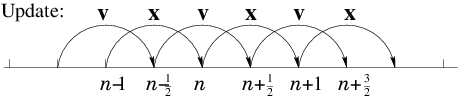Figure 2.6: Staggered values for $x$ and $v$ are updated leap-frog style.
One trap for the unwary in a Leap-Frog scheme is the specification of initial values. If we want to calculate an orbit which has specified initial position ${\mathbit{x}}_{0}$ and velocity ${\mathbit{v}}_{0}$ at time $t={t}_{0}$, then it is not sufficient simply to put the velocity initially equal to the specified ${\mathbit{v}}_{0}$. That is because the first velocity, which governs the position step from ${t}_{0}$ to ${t}_{1}$ is not ${\mathbit{v}}_{0}$, but ${\mathbit{v}}_{1/2}$. To start the integration off correctly, therefore, we must take a half-step in velocity by putting ${\mathbit{v}}_{1/2}={\mathbit{v}}_{0}+{\mathbit{a}}_{0}\left({t}_{1}-{t}_{0}\right)/2$, before beginning the standard integration.
Leap-Frog schemes generally possess important conservation properties such as conservation of momentum, that can be essential for realistic simulation with a large number of particles.

## Worked Example: Stability of finite differences

Find the stability limits when solving by discrete finite differences of length $\mathit{\Delta }$, using explicit or implicit schemes, the initial value equation
 $\frac{{d}^{2}y}{{\mathit{dx}}^{2}}+2\mathit{\alpha }\frac{\mathit{dy}}{\mathit{dx}}+{k}^{2}y=g\left(x\right),$ $\left(2.26\right)$
where $\mathit{\alpha }$ is a positive constant $<|k|$.

First, reduce the equation to standard first-order form by writing
 $\frac{d}{\mathit{dx}}\left(\begin{array}{c}\hfill {y}^{\left(0\right)}\hfill \\ \hfill {y}^{\left(1\right)}\hfill \end{array}\right)=\left(\begin{array}{c}\hfill {y}^{\left(1\right)}\hfill \\ \hfill g\left(x\right)-2\mathit{\alpha }{y}^{\left(1\right)}-{k}^{2}{y}^{\left(0\right)}\hfill \end{array}\right)=\left(\begin{array}{cc}\hfill 0\hfill & \hfill 1\hfill \\ \hfill -{k}^{2}\hfill & \hfill -2\mathit{\alpha }\hfill \end{array}\right)\left(\begin{array}{c}\hfill {y}^{\left(0\right)}\hfill \\ \hfill {y}^{\left(1\right)}\hfill \end{array}\right)+\left(\begin{array}{c}\hfill 0\hfill \\ \hfill g\left(x\right)\hfill \end{array}\right).$ $\left(2.27\right)$
Now, notice that, in either form, this equation has a sort of driving term $g\left(x\right)$ independent of $y$. It can be removed from the stability analysis by considering a new variable $z$ which is the difference between the finite difference numerical solution that we are trying to analyse, ${y}_{n}\left(x\right)$, and the exact solution of the differential equation, $y\left(x\right)$. Thus ${z}_{n}={y}_{n}-y\left({x}_{n}\right)$. The quantity $z$ satisfies a discretized form of the homogeneous version of eq. (2.26); with the term $g\left(x\right)$ removed. Consequently analysing that homogeneous equation tells us whether the difference $z$ between the numerical and the exact solutions is stable or not. That is what we really want to know. For stability, we pay no attention to $g\left(x\right)$. The resulting homogeneous equation is of exactly the same form as eq. (2.24): $\frac{d}{\mathit{dx}}\mathbf{z}=\mathbf{A}\mathbf{z}$. Each independent solution for $\mathbf{z}$ is thus an eigenvector of the matrix $\mathbf{A}$ such that $\frac{d}{\mathit{dx}}\mathbf{z}=\mathit{\lambda }\mathbf{z}$ . The eigenvalue $\mathit{\lambda }$ decides its stability. An explicit (Euler) scheme of step length $\mathit{\Delta }$ will result in a step amplification factor, such that ${\mathbf{z}}_{n+1}=F{\mathbf{z}}_{n}$ with $F=1+\mathit{\lambda }\mathit{\Delta }$, and will be unstable if $|F|>1$.
The eigenvalues of $\mathbf{A}$ satisfy

 $0=|\begin{array}{cc}\hfill -\mathit{\lambda }\hfill & \hfill 1\hfill \\ \hfill -{k}^{2}\hfill & \hfill -\mathit{\lambda }-2\mathit{\alpha }\hfill \end{array}|={\mathit{\lambda }}^{2}+2\mathit{\alpha }\mathit{\lambda }+{k}^{2},$ $\left(2.28\right)$
with solutions
 $\mathit{\lambda }=-\mathit{\alpha }±\sqrt{{\mathit{\alpha }}^{2}-{k}^{2}}.$ $\left(2.29\right)$
These solutions are complex if ${k}^{2}>{\mathit{\alpha }}^{2}$, in which case,
 $|F{|}^{2}=\left(1-\mathit{\alpha }\mathit{\Delta }{\right)}^{2}+\left({k}^{2}-{\mathit{\alpha }}^{2}\right){\mathit{\Delta }}^{2}=1-2\mathit{\alpha }\mathit{\Delta }+{k}^{2}{\mathit{\Delta }}^{2}.$ $\left(2.30\right)$
$|F|$ is greater than unity unless $\mathit{\Delta }<2\mathit{\alpha }/{k}^{2}$, which is the stability criterion of the Euler scheme. If $\mathit{\alpha }=0$, so that the homogeneous equation is an undamped harmonic oscillator, the explicit scheme is unstable for any $\mathit{\Delta }$. A fully implicit scheme, by contrast, has $F={\mathbf{z}}_{n+1}/{\mathbf{z}}_{n}=1/\left(1-\mathit{\lambda }\mathit{\Delta }\right)$, for which $|F{|}^{2}=1/\left(1+2\mathit{\alpha }\mathit{\Delta }+{k}^{2}{\mathit{\Delta }}^{2}\right)$, always less than one. The implicit scheme is always stable.

## Exercise 2. Integrating Ordinary Differential Equations.

1. Reduce the following higher-order ordinary differential equations to first-order vector differential equations, which you should write out in vector format.
(a) $\frac{{d}^{2}y}{{\mathit{dt}}^{2}}=-1$
(b) $Ay+B\frac{\mathit{dy}}{\mathit{dx}}+C\frac{{d}^{2}y}{{\mathit{dx}}^{2}}+D\frac{{d}^{3}y}{{\mathit{dx}}^{2}}=E$
(c) $\frac{{d}^{2}y}{{\mathit{dx}}^{2}}=2{\left(\frac{\mathit{dy}}{\mathit{dx}}\right)}^{2}-{y}^{3}$

2. Accuracy order of ODE schemes. For notational convenience, we start at $x=y=0$ and consider a small step in $x$ and $y$ of the ODE $\mathit{dy}/\mathit{dx}=f\left(y,x\right)$. The Taylor expansion of the derivative function along the orbit is
 $f\left(y\left(x\right),x\right)={f}_{0}+\frac{{\mathit{df}}_{0}}{\mathit{dx}}x+\frac{{d}^{2}{f}_{0}}{{\mathit{dx}}^{2}}\frac{{x}^{2}}{2!}+\dots$ $\left(2.31\right)$

 (a) Integrate $\frac{\mathit{dy}}{\mathit{dx}}=f\left(y\left(x\right),x\right)$ term by term to find the solution for $y$ to third-order in $x$. (b) Suppose ${y}_{1}={f}_{0}x$. Find ${y}_{1}-y\left(x\right)$ to second-order in $x$. (c) Now consider ${y}_{2}=f\left({y}_{1},x\right)x,$ show that it is equal to $f\left(y,x\right)x$ plus a term that is third-order in $x$. (d) Hence find ${y}_{2}-y$ to second-order in $x$. (e) Finally show that ${y}_{3}=\frac{1}{2}\left({y}_{1}+{y}_{2}\right)$ is equal to $y$ accurate to second-order in $x$.
[Comment. The third-order term in part (c) involves the partial derivative $\partial f/\partial y$ rather than the derivative along the orbit. Proving rigorously that the fourth-order Runge Kutta scheme really is fourth-order, is rather difficult because it requires keeping track of such partial derivatives.]
Programming Exercise
Write a program to integrate numerically from $t=0$ to $t=4/\mathit{\omega }$ the ODE
 $\frac{\mathit{dy}}{\mathit{dt}}=-\mathit{\omega }y$

with $\mathit{\omega }$ a positive constant, starting from $y\left(0\right)=1$, proceeding as follows.
(a) Use the explicit Euler scheme
 ${y}_{n+1}={y}_{n}-\mathit{\Delta }t\mathit{\omega }{y}_{n}.$

(b) Use the implicit scheme
 ${y}_{n+1}={y}_{n}-\mathit{\Delta }t\mathit{\omega }{y}_{n+1}.$

In each case, find numerically the fractional error at $t=4/\mathit{\omega }$ for the following choices of timestep.
(i) $\mathit{\omega }\mathit{\Delta }t=0.1$ (ii) $\mathit{\omega }\mathit{\Delta }t=0.01$ (iii) $\mathit{\omega }\mathit{\Delta }t=1$

(c) Find experimentally the timestep value at which the explicit scheme becomes unstable. Verify that the implicit scheme never becomes unstable.